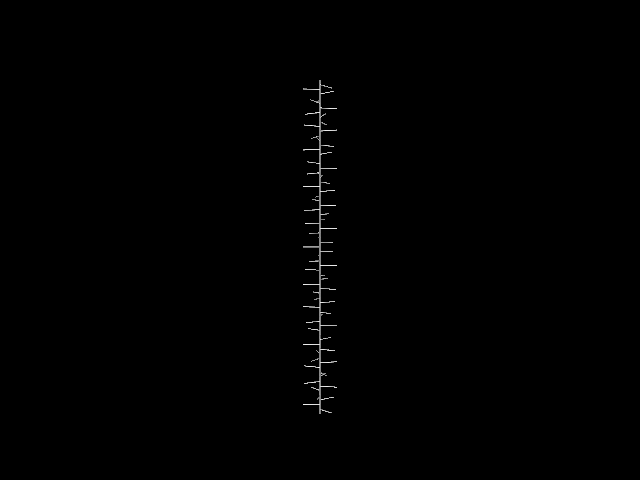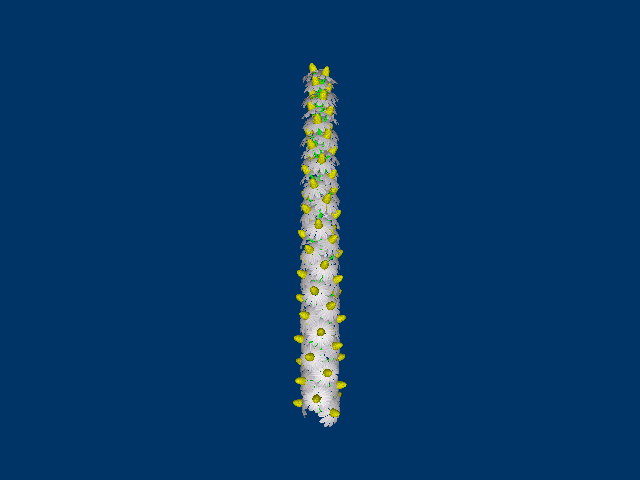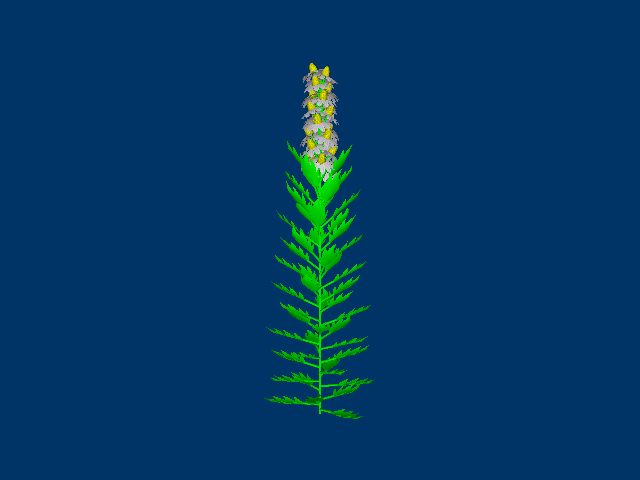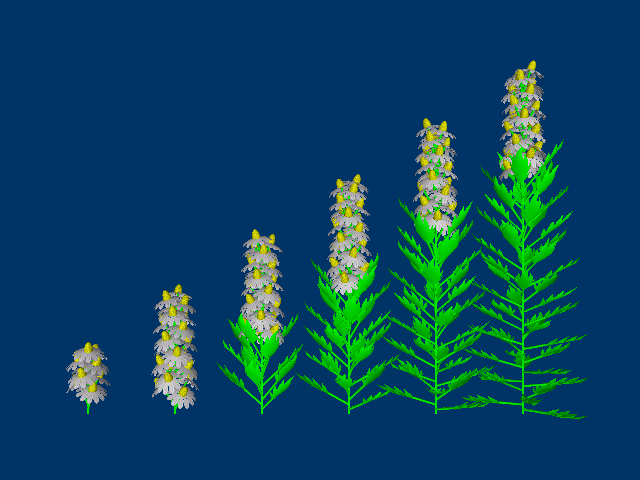# Parametric L-systems

(back to contents)

Parametric L-systems have rules with parameters. Technically all L-systems in the previous chapters are parametric because they have rules like `forward(10)` and `turn(30)`, but all of those rules were already predefined. This chapter explains how to define custom rules with parameters and also how to associate code with a rule that turtle can later execute.```(with-graphics (iteration-count 72) (set-axiom (apex)) (add-rule (apex -> F(8) apex))) ```

This simple example contains `apex` rule that does no more than slowly grow the stem of a plant longer and longer.```(defconstant +fibonacci+ 137.50777) (with-graphics (iteration-count 72) (set-axiom (apex)) (add-rule (apex -> F(8) roll(+fibonacci+) branch apex)) (add-rule (branch -> [ pitch(90) F(30) ]))) ```

Next, there are some side branches added at a straight angle from the stem. Each new side branch is rotated (rolled) around the stem axis by the Fibonacci angle (or the golden angle). Fibonacci angle is a full, 360 degree angle divided by the golden ratio squared. Fibonacci angle often appears throughout nature, most commonly in a phenomenon called phyllotaxis.```(defconstant +fibonacci+ 137.50777) (with-graphics (iteration-count 72) (set-axiom (apex)) (add-option bend (turtle-pitch x)) (add-rule (apex -> F(8) roll(+fibonacci+) branch apex)) (add-rule (bend(x) -> bend((+ x 1.0)))) (add-rule (branch -> [ bend(18) F(30) ]))) ```

In this example, a simple parametric rule `bend` is defined. The `bend` rule is similar to `pitch` except that in each iteration it is rewritten with the previous angle increased by one degree. That is the reason why "new" side branches form a steeper angle with the stem than the "old" side branches. The general syntax of a parametric rule definition is the rule name followed by a list of parameters (list meaning a lisp list: elements in braces separated by whitespace), after which comes the arrow (`->`) followed by rule expansion. Rule parameters can be used in arbitrary lisp s-expressions to form arguments for rules in expansion.

When the axiom is rewritten "iteration count" number of times, turtle goes through one final production and calls the drawing code associated with each rule. Drawing code for a custom rule can be added with `add-option` macro. This macro takes two arguments: rule name and lisp code form. For example, in the program there is a predefined lisp function that rotates turtle around its local X axis:

```(defun turtle-pitch (angle)
...)
```

Now, if there wasn't a predefined rule called `pitch`, it could be implemented by the following lines:

```(add-option pitch (turtle-pitch angle))
``````(defconstant +fibonacci+ 137.50777) (defparameter *green* (create-color 0.0 1.0 0.0)) (with-graphics (iteration-count 72) (set-background-color (create-color 0.0 0.2 0.4)) (set-axiom (radius(2) color(*green*) apex)) (add-option bend (turtle-pitch x)) (add-rule (apex -> F(8) roll(+fibonacci+) branch apex)) (add-rule (bend(x) -> bend((+ x 1.0)))) (add-rule (branch -> [ bend(18) F(30) ]))) ```

Just to add some decorations to make examples look more consistent with the ones in previous chapters.```(defconstant +fibonacci+ 137.50777) (defparameter *green* (create-color 0.0 1.0 0.0)) (with-graphics (iteration-count 72) (set-background-color (create-color 0.0 0.2 0.4)) (set-axiom (radius(2) color(*green*) apex)) (add-option bend (turtle-pitch x)) (add-rule (apex -> F(8) roll(+fibonacci+) branch apex)) (add-rule (bend(x) -> bend((+ x 1.0)))) (add-rule (branch -> [ bend(18) organ ])) (add-rule (organ -> F(30) obj("blossom" 7.0))) (read-wavefront "blossom.obj" "blossom.png")) ```

This example adds some blossoms at the end of branches.```(defconstant +fibonacci+ 137.50777) (defparameter *green* (create-color 0.0 1.0 0.0)) (with-graphics (iteration-count 72) (set-background-color (create-color 0.0 0.2 0.4)) (set-axiom (radius(2) color(*green*) apex)) (add-option bend (turtle-pitch x)) (add-option blossom (turtle-object "blossom" 7.0)) (add-option leaf (turtle-object "leaf" 7.0 *green*)) (add-rule (apex -> F(8) roll(+fibonacci+) branch apex)) (add-rule (bend(x) -> bend((+ x 1.0)))) (add-rule (branch -> [ bend(18) organ ])) (add-rule (organ -> F(30) blossom(20))) (add-rule ((blossom(x) ? (> x 0) -> blossom((1- x))) (blossom(x) ? (= x 0) -> roll(-90) leaf))) (add-macro (leaf -> obj("leaf" 10.0 *green*))) (read-wavefront "blossom.obj" "blossom.png") (read-wavefront "leaf.obj")) ```

One more feature that goes together with parametric rules is conditionals. Instead of just writing rules in braces, one more level of parenthesis can be added, thus making it possible to expand a single rule in multiple ways. In that case each rule definition can contain `?` sign followed by test form placed after parameter list. This particular example contains such a rule called `blossom`. This rule draws a blossom, but after twenty iterations turns itself into a leaf.

Parametric rules with conditionals are a convenient way to implement stochastic L-systems. Consider as an example that somebody gathered statistics on certain species of some trees. From all the buds that appear in the spring, 20% are eaten by bugs, 50% turn into leaves, and the remaining 30% turn into flowers. Well, such statistics can be expressed nicely with the following rule:

```(add-macro (bud -> r-bud((random 100)))
(add-rule ((r-bud(x) ? (< x 20) -> )
(r-bud(x) ? (< x 70) -> leaf)
(r-bud(x) ? (< x 100) -> flower)))
``````(defconstant +fibonacci+ 137.50777) (defparameter *green* (create-color 0.0 1.0 0.0)) (with-graphics (iteration-count 72) (set-background-color (create-color 0.0 0.2 0.4)) (add-macro (right -> move((create-point 350.0 0.0 0.0)))) (set-axiom (radius(2) color(*green*) right X)) (add-rule (X -> [ apex ] turn(-90) jump(150) turn(90) delay(10))) (add-rule ((delay(x) ? (> x 0) -> delay((1- x))) (delay(x) ? (= x 0) -> X))) (add-option bend (turtle-pitch x)) (add-option blossom (turtle-object "blossom" 7.0)) (add-option leaf (turtle-object "leaf" 7.0 *green*)) (add-rule (apex -> F(8) roll(+fibonacci+) branch apex)) (add-rule (bend(x) -> bend((+ x 1.0)))) (add-rule (branch -> [ bend(18) organ ])) (add-rule (organ -> F(40) blossom(20))) (add-rule ((blossom(x) ? (> x 0) -> blossom((1- x))) (blossom(x) ? (= x 0) -> roll(-90) leaf))) (add-macro (leaf -> obj("leaf" 10.0 *green*))) (read-wavefront "blossom.obj" "blossom.png") (read-wavefront "leaf.obj")) ```

To illustrate the development of this plant, "row of plants" rule `X` is used. It's just that this time it must be a little bit more elaborate than in the previous chapter, because now the L-system has 72 iterations. So `delay`, a parametric rule with conditionals, is introduced. It is very similar to `blossom` rule which in essence delays creation of the leaf, while `delay` delays creation of the next plant.

(back to contents)